Electrical machines and torsional vibrations - stability

Hi there! I’ve indeed written quite a bit about vibrations in electrical machines recently, and this post does not break the rule. We began by looking at the different types of vibrations, and last time we took a closer look on torsional vibrations.

This time, we’ll be continuing on a very related topic. Remember how torsional resonances were generally a bad thing? Good if you do, because they are.

But for resonances to be catastrophic, something has to excite them. And for that role, the electrical machine is a good candidate. Its torque is never perfectly smooth, after all.

However, one defining characteristic of the machines is that their torque depends on the angle of the rotor. And that’s precisely what will be varying when torsional vibrations occur.

To rephrase: let’s say we have some torsional oscillations going on. These oscillations will influence the torque production of the machine. In the good case, the interaction is such that the oscillations are damped. In the bad case, not.

So, it seems that we’ll have to include the magnetics of the machine to our mechanical analysis. Sounds complex, right?

Well luckily, there’s a handy shortcut. Enter the frequency response function.

### FRF

The frequency response function – or FRF for short – is a handy little fellow. As I’ve written before, the idea of FRF is quite simple. We add ripple to the rotor position, and measure (or compute) how the torque produced by the machine varies as response.

Expressed mathematically, let’s say the angle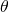of the rotor is fixed to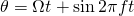.

Here, the first term corresponds to the constant-speed rotation. The second-term is then a unit-amplitude oscillation term at the frequency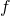.

Next, we determine the part of the electromagnetic torque that also oscillates at the frequency, and that’s pretty much our FRF.

##### Some details

Well, not exactly. The torque variation might not be a pure sine function – it might have a cosine component, too. In other words, we may have a phase shift between the input and the output.

For that reason, the FRF is defined with the Fourier transform. You should be familiar with it, so let’s just say the Fourier transform is a way of dividing any signal into components oscillating at different frequencies. The original signal is then the sum of those components (mathematical exceptions aside).

With this in mind, it’s easy to see how the transform relates to the FRF. Simply, we take the Fourier transform of the torque, and divide it by the Fourier transform of the rotation angle. Thus, we get a complex-valued function, i.e. something with real and imaginary parts.

##### Interpretation of the FRF

Alright, now that we’ve wrapped our minds around the FRF, let’s move on to a curious equivalence.

Indeed, the let’s say that we have a purely mechanical spring-damper system. If we compute the FRF of that system, we can show that the real part of the function corresponds to the spring coefficient. Vice versa, the negative imaginary part corresponds to the damping.

Thus, we can conclude the following: At any torsional oscillation frequency, the electrical machine can be replaced by a spring-damper system. The spring coefficient is set equal to the imaginary part of the FRF at that frequency; while the damping is obtained by multiplying the imaginary part by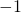.

### Implications

Got lost in the mathematics? No worries.

The takeaway is simple: For analyzing torsional vibrations, the electrical machine can be replaced by a simple torsion spring and a damper.

Sounds good, doesn’t it?

##### Instability

Below, we can see an example of the FRF function.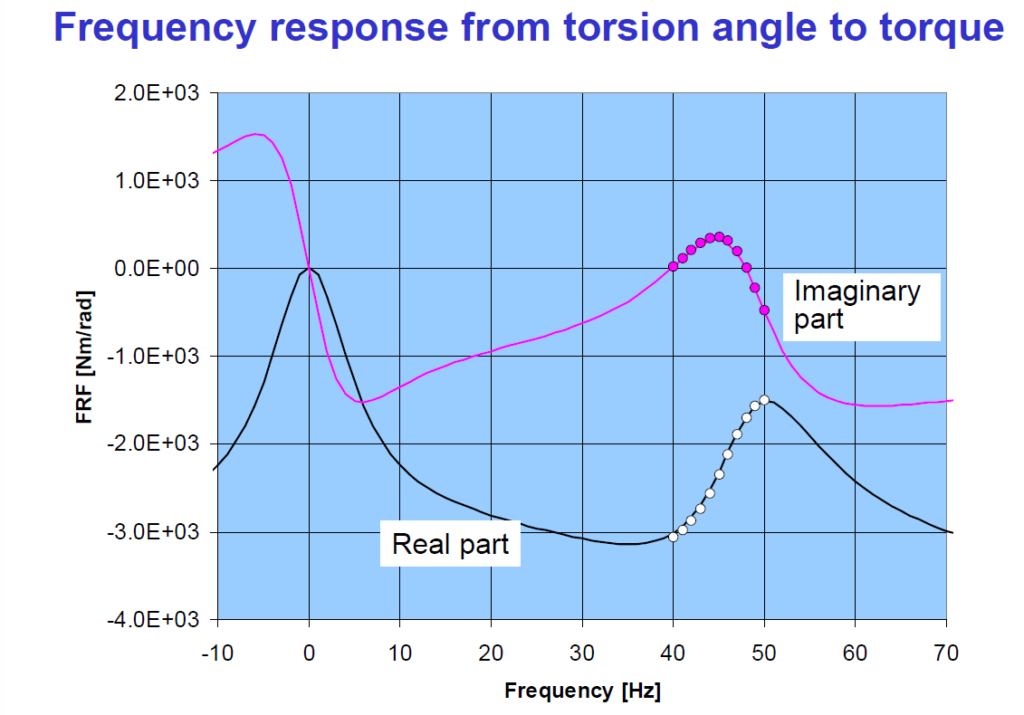Now, remember this: damping = minus imaginary part. Now, look again at the figure, at frequencies 40-47 Hz or so. You see that the imaginary part is positive.

Thus, at these frequencies, the electrical machine acts like a spring with negative damping. In other words, it’ll start feeding energy into oscillations at this frequency.

Generally, that’s not a good thing. This is doubly true whenever the mechanical system has a natural frequency somewhere near this range.

##### Limitations

Obviously, there are some limitations to this approach. First and foremost, the FRF is only valid for small-amplitude oscillations. If they get larger, the nonlinearity of the machine steps in, and the relationship between angle and torque gets more complex.

On the other hand, the shaft would usually break before that. So most of the time, the FRF remains a valid tool.

### Conclusion

• For small-amplitude torsional vibrations, an electrical machine behaves like a spring-damper system.
• The spring constant and damping coefficient can be obtained from the frequency-response function (FRF) of the machine.
• The electromechanical system may be unstable, with the machine feeding energy to the vibrations.

-Antti

Need help with electric motor design or design software? Let's get in touch - satisfaction guaranteed!Stability of Torsional Vibrations